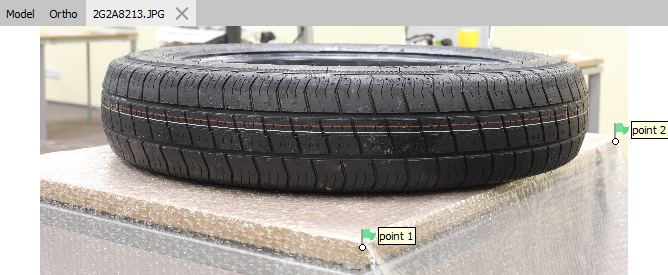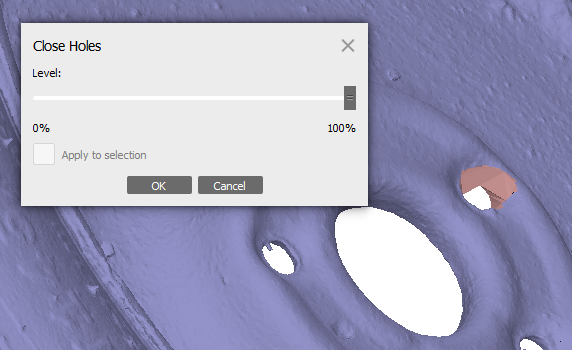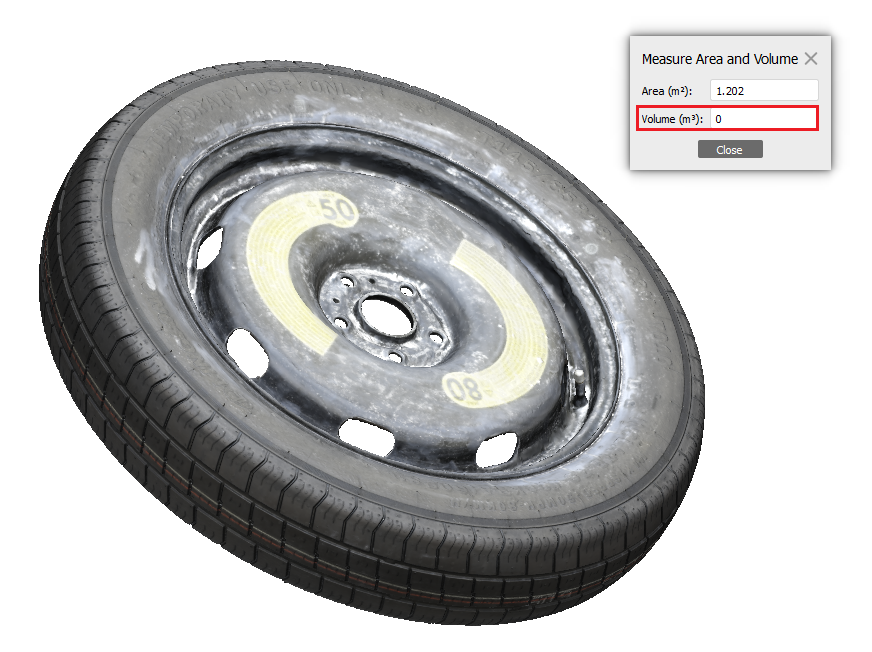This tutorial illustrates how to perform volume measurements based on 3D mesh model in Metashape Professionaedition. The following steps are to be performed for the volume measurement:

## Scaling model

First of all please make sure that the model is georeferenced or scaled. You may find useful information on the model georeferencing and scaling in the following articles:

3D model reconstruction

In the example project the model is scaled using scale bars. A scale bar is basically a known distance on the model. The distance can be set manually, for example, the length of one side of the table was measured and assigned as distance between point 1 and point 2 on the model.`A detailed description of the steps to create a scale bar can be found in our article:  Creating scale bars in the project without coded targets. `

Once the model is scaled you may proceed to the next step## Close Holes

In Metashape the volume can be calculated only for models with closed geometry. To close the holes in the model geometry use the corresponding tool in Tools > Mesh > Close Holes. In the Close Holes dialog drag slider to the right end position. Click OK button to initiate the procedure.

Additional polygons will be generated to cover all holes in the model geometry, on the illustration below the new faces are colored in pink:## Measure Volume

Once the preparation steps are completed the volume of the model can be measured with a single-click operation. Select Measure Area and Volume... command from Tools menu. The Measure Area and Volume dialog window will appear, the values in cubic meters for volume and in square meters for area will be displayed:`If the model has holes (and close holes procedure has not been performed), the volume values will not be calculated (the value will be 0), see the illustration below``The Area is calculated as the sum of the areas of all polygons of the mesh.`